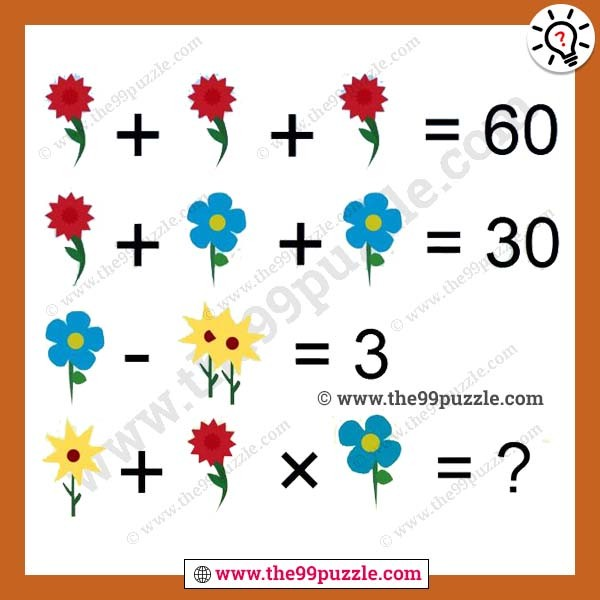# Flower Math Equation Picture Puzzle for Students with Answer – Puzz108

Solve the Flower Math Equation Picture Puzzle. In this Picture Puzzles, there are Maths Equations given with the flower. Can you solve these Maths Equations to find the value of each of these flowers and then solve the last question to find the number which will replace the question mark?###### Explanation:

Red flower=20

Blue flower= 5 (Petals)

Yellow flower = 1

20+20+20=60

20+5+5=30

5−(1+1)=3

1+20×4 (4 petals)= 81